Python 与 Excel 不得不说的事

xlrd、xlwt、xlutils

• xlrd － 读取 Excel 文件

• xlwt － 写入 Excel 文件

• xlutils － 操作 Excel 文件的实用工具，如复制、分割、筛选等

（更新：有人提到另外几个 Python 处理 Excel 的库，有些的确会比 python-excel 系列好用。之后会再做一篇对比，敬请期待。

## 读取

```import xlrd
# 打开 xls 文件
book = xlrd.open_workbook("test.xls")
print "表单数量:", book.nsheets
print "表单名称:", book.sheet_names()
# 获取第1个表单
sh = book.sheet_by_index(0)
print u"表单 %s 共 %d 行 %d 列" % (sh.name, sh.nrows, sh.ncols)
print "第二行第三列:", sh.cell_value(1, 2)
# 遍历所有表单
for s in book.sheets():
for r in range(s.nrows):
# 输出指定行
print s.row(r)```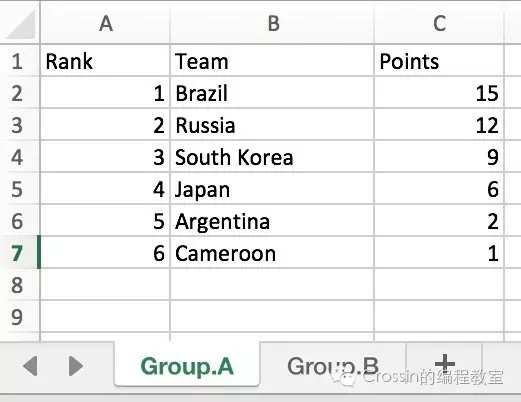[text:u'Rank', text:u'Team', text:u'Points']
[number:1.0, text:u'Brazil', number:15.0]
[number:2.0, text:u'Russia', number:12.0]
...

• open_workbook 打开文件

• sheet_by_index 获取某一个表单

• sheets 获取所有表单

• cell_value 获取指定单元格的数据

## 写入

```import xlwt
# 创建 xls 文件对象
wb = xlwt.Workbook()
# 新增一个表单
# 按位置添加数据
sh.write(0, 0, 1234.56)
sh.write(1, 0, 8888)
sh.write(2, 0, 'hello')
sh.write(2, 1, 'world')
# 保存文件
wb.save('example.xls')```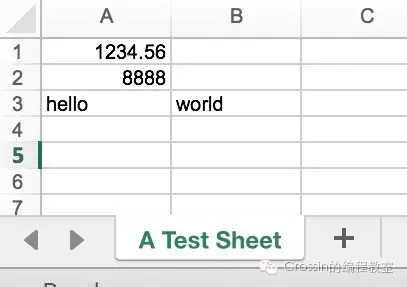• Workbook 创建文件对象

• write 在指定单元格写入数据

## 修改

```from xlrd import open_workbook
from xlutils.copy import copy
# 打开文件
rb = open_workbook("example.xls")
# 复制
wb = copy(rb)
# 选取表单
s = wb.get_sheet(0)
# 写入数据
s.write(0, 1, 'new data')
# 保存
wb.save('example.xls')```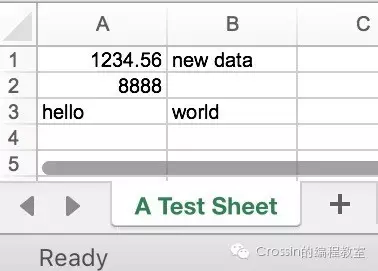## 时间转换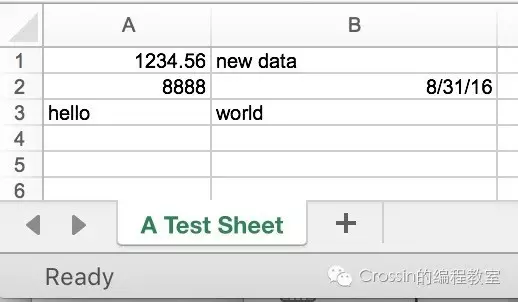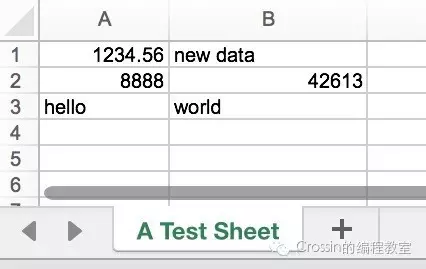[number:8888.0, xldate:42613.0]

`new_date = xlrd.xldate.xldate_as_datetime(date, book.datemode)`

date 是对应单元格的数据，book 是打开的文件对象。

`xlrd.xldate.xldate_from_datetime_tuple`

```style = xlwt.easyxf(num_format_str='D-MMM-YY')
ws.write(1, 0, datetime.now(), style)```

RTFMSTFW